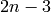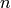# Gauss-Lobatto integration with adaptive refinement¶

The Gauss-Lobatto quadrature is an alternative to the widely used Gaussian quadrature that is exact for polynomials of orderwhereis the number of points used.

Compared to the standard Gaussian quadrature, the Lobatto rules have the advantage that the end-points of the interval to be integrated are included in the points to be evaluated. This means adaptive refinement is easy to implement and understand in this scheme.

## Outline of the algorithm¶

The algorithm broadly consists of recursively evaluating a 7-point Lobatto quadrature rule.

At each recursion level, the 7- point and 4-point Lobatto quadratures are estimated. If these estimates are close enough, it is assumed that the required tolerance has been met and the this recursion level returns the value of the 7-point estimate and does not cause further processing.

If the two estimates are sufficiently different, the interval to be integrated is split into six sub-intervals, with the boundaries of these intervals defined by the 7 points used in the quadrature (therefore re-using all of the function evaluations). The same procedure is then applied to these six sub-intervals and the result of the current recursion level is the sum of results of the six sub-intervals.

## Implementation¶

The implementation shown below is closely based on the open source implementation in the QuantLib library. For the purposes of this article I have made the implementation entirely free-standing and simplified it slightly by removing the relative tolerance stopping criterion.

The implementation is quite straightforward, with only a few points to note:

1. Traditional stack-based recursion is used. Very deep integrations could therefore exhaust the process stack even though plenty of actual and virtual memory remain. In these cases the stack limits should be adjusted accordingly or the implementation modified to use a separate stack-like area.
2. Function to be integrated is represented by the boost::function objects (documentation). This allows easy use of both plain functions and functor classes
3. The code for checking if the absolute value of estimated error is less than the required tolerance makes use of the finite precision of floating point numbers stored in 64 bit fields. For this reason the value std::numeric_limits<double>::epsilon() is used in the program (see this article)

## The code¶

```/*
Copyright (C) 2010 Bojan Nikolic <bojan@bnikolic.co.uk>

Portions originaly part of QuantLib http://quantlib.org/

This program is distributed in the hope that it will be useful, but WITHOUT
ANY WARRANTY; without even the implied warranty of MERCHANTABILITY or FITNESS
FOR A PARTICULAR PURPOSE.

*/
#include <cmath>
#include <boost/function.hpp>

#include <iostream>
#include <boost/format.hpp>

/** \brief Perform a single step of the Gauss-Lobatto integration

\param f Function to integrate
\param a Lower integration limit
\param b Upper integration limit

\param fa Value of function at the lower limit (used to save an
evaluation when refinement is used)

\param fa Value of function at the upper limit (used to save an
evaluation when refinement is used)

\param neval Number of evaluations made so far

\param maxeval Maximum number of evalutions which should not be
exceeded

\param acc Required accuracy expressed in units of
std::numeric_limits<double>::epsilon(). This allows less-than
comparison by using addition and equality.
*/
double GaussLobattoIntStep(const boost::function<double (double)>& f,
double a, double b,
double fa, double fb,
size_t &neval,
size_t maxeval,
double acc)
{

// Constants used in the algorithm
const double alpha = std::sqrt(2.0/3.0);
const double beta  = 1.0/std::sqrt(5.0);

if (neval >= maxeval)
{
throw std::runtime_error("Maximum number of evaluations reached in GaussLobatto");
}

// Here the abcissa points and function values for both the 4-point
// and the 7-point rule are calculated (the points at the end of
// interval come from the function call, i.e., fa and fb. Also note
// the 7-point rule re-uses all the points of the 4-point rule.)
const double h=(b-a)/2;
const double m=(a+b)/2;

const double mll=m-alpha*h;
const double ml =m-beta*h;
const double mr =m+beta*h;
const double mrr=m+alpha*h;

const double fmll= f(mll);
const double fml = f(ml);
const double fm  = f(m);
const double fmr = f(mr);
const double fmrr= f(mrr);
neval+=5;

// Both the 4-point and 7-point rule integrals are evaluted
const double integral2=(h/6)*(fa+fb+5*(fml+fmr));
const double integral1=(h/1470)*(77*(fa+fb)
+432*(fmll+fmrr)+625*(fml+fmr)+672*fm);

// The difference betwen the 4-point and 7-point integrals is the
// estimate of the accuracy
const double estacc=(integral1-integral2);

// The volatile keyword should prevent the floating point
// destination value from being stored in extended precision
// registers which actually have a very different
// std::numeric_limits<double>::epsilon().
volatile double dist = acc + estacc;

if(dist==acc || mll<=a || b<=mrr)
{
if (not (m>a && b>m))
{
throw std::runtime_error("Integration reached an interval with no more machine numbers");
}
return integral1;
}
else {
return  GaussLobattoIntStep(f, a, mll, fa, fmll, neval, maxeval, acc)
+ GaussLobattoIntStep(f, mll, ml, fmll, fml, neval, maxeval, acc)
+ GaussLobattoIntStep(f, ml, m, fml, fm, neval, maxeval, acc)
+ GaussLobattoIntStep(f, m, mr, fm, fmr, neval, maxeval, acc)
+ GaussLobattoIntStep(f, mr, mrr, fmr, fmrr, neval, maxeval, acc)
+ GaussLobattoIntStep(f, mrr, b, fmrr, fb, neval, maxeval, acc);

}
}

/** \brief Compute the Gauss-Lobatto integral

\param f The function to be integrated

\param a The lower integration limit

\param b The upper integration limit

\param abstol Absolute tolerance -- integration stops when the
error estimate is smaller than this

\param maxeval Maxium of evaluations to make. If this number of
evalution is made without reaching the requied accuracy, an
exception of type std::runtime_error is thrown.
*/
double GaussLobattoInt(const boost::function<double (double)>& f,
double a, double b,
double abstol,
size_t maxeval)
{
const double tol_epsunit=abstol/std::numeric_limits<double>::epsilon();
size_t neval=0;
return GaussLobattoIntStep(f, a, b,
f(a), f(b),
neval,
maxeval,
tol_epsunit);
}

// Below is a very simple illustration of the code

double samplef(double x)
{
return std::sin(x);
}

int main(void)
{
double res=GaussLobattoInt(&samplef,
0, 10,
1e-10,
1000);
std::cout<<boost::format("Result is %g ") %  res
<<std::endl;

}
```